## 程序员硬核操作 | 利用代码程序建立新冠病毒传播模型

### 文章目录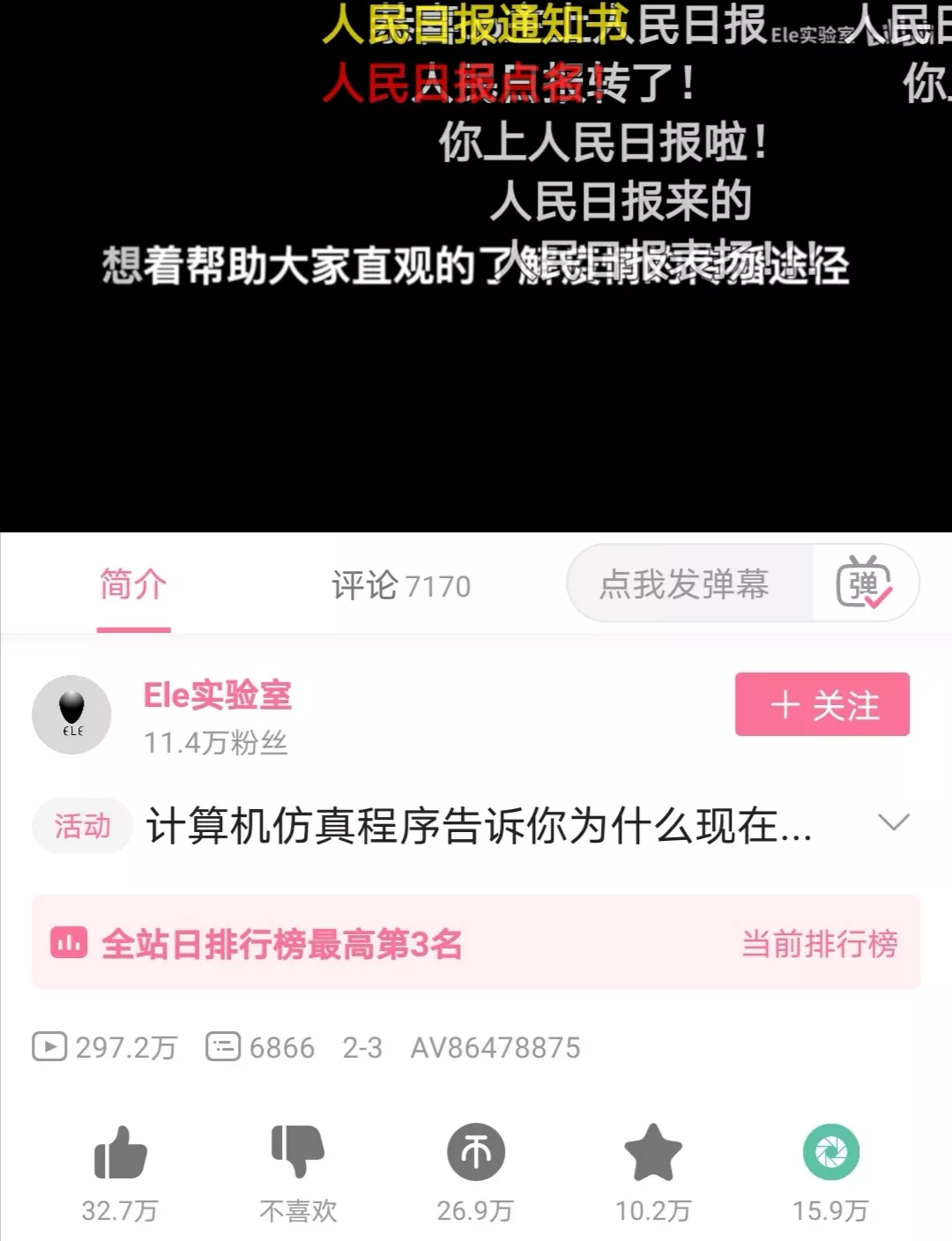https://www.bilibili.com/video/av86478875

# 一、JFrame面板组件布局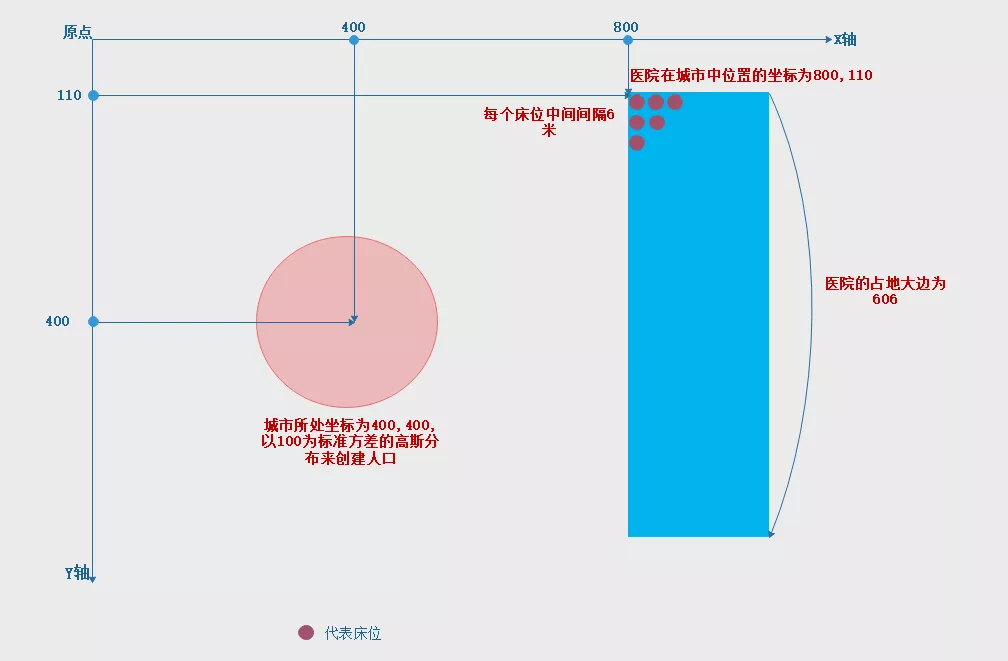# 二、数学概念：高斯（正态）分布

## 2.1 高斯分布概念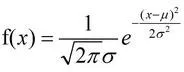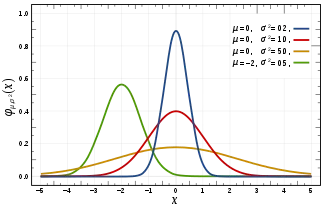## 2.2 正态变量的标准化

v 为服从高斯分布的数据

σ 为标准差

μ 为平均值

W 对v进行标准化处理后的数据，依然是服从高斯分布的

W = (V - μ) / σ

V = W * σ + μ

## 2.3 高斯分布在Java中的应用

java.util.Random函数有个方法叫做nextGaussian()函数，定义如下：

``````/*

* @return the next pseudorandom, Gaussian ("normally") distributed

* {@code double} value with mean {@code 0.0} and

* standard deviation {@code 1.0} from this random number

* generator's sequence

*/

synchronized public double nextGaussian() {

// See Knuth, ACP, Section 3.4.1 Algorithm C. if (haveNextNextGaussian) {

haveNextNextGaussian = false; return nextNextGaussian;

} else {

double v1, v2, s;

do {

v1 = 2 * nextDouble() - 1; // between -1 and 1

v2 = 2 * nextDouble() - 1; // between -1 and 1 s = v1 * v1 + v2 * v2;

} while (s >= 1 || s == 0);

double multiplier = StrictMath.sqrt(-2 * StrictMath.log(s)/s); nextNextGaussian = v2 * multiplier;

haveNextNextGaussian = true;

return v1 * multiplier;

}

}
``````

``````double value = sigma * new Random().nextGaussian() + 0.99;
``````

# 三、核心代码解读

## 3.1启动类函数：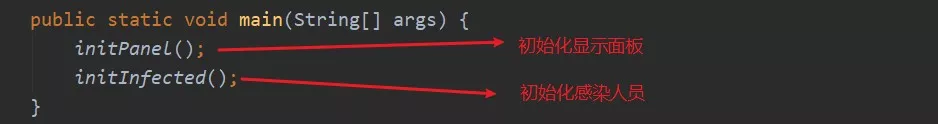## 3.2画布相关代码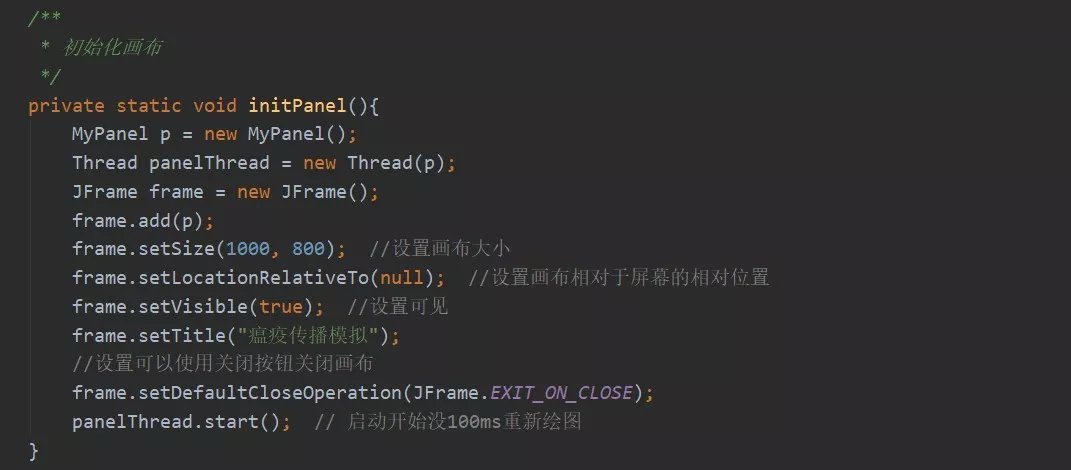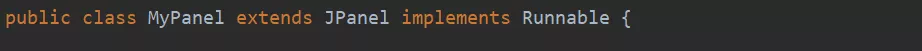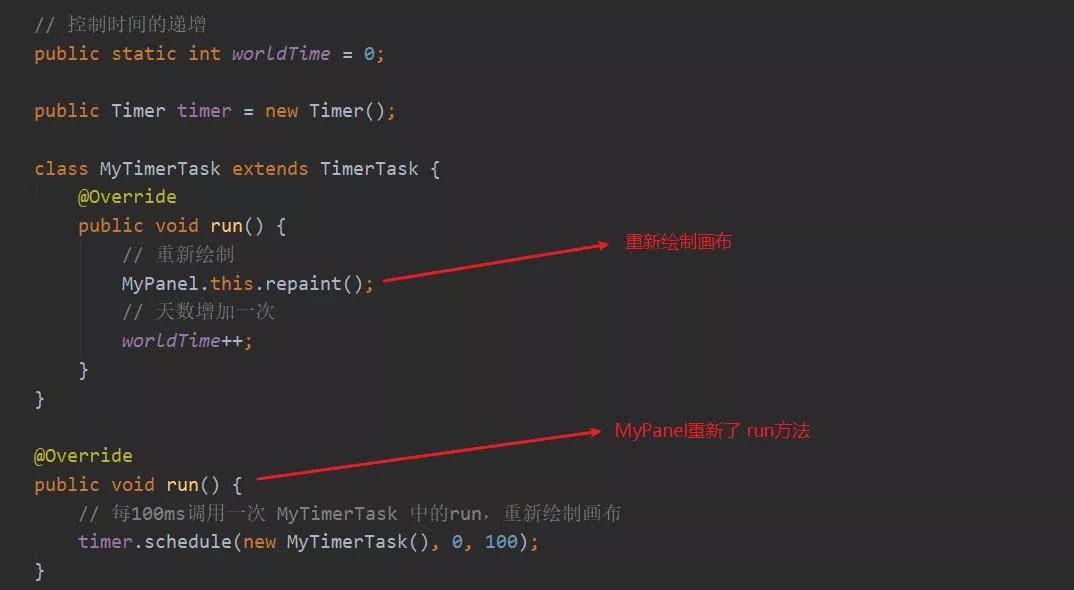## 3.3初始化感染人员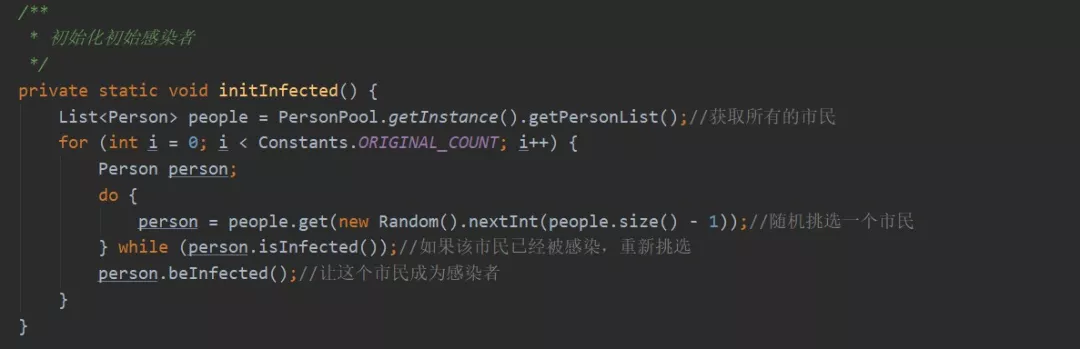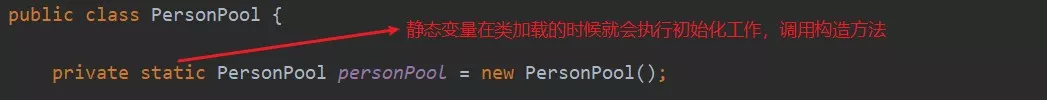PersonPool的构造函数：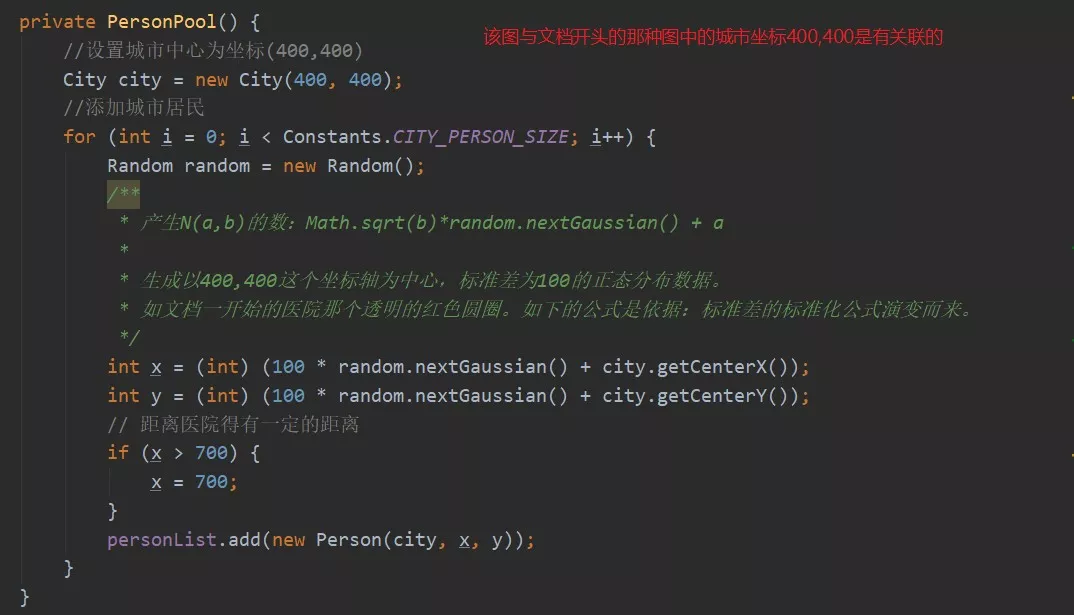## 3.4Person类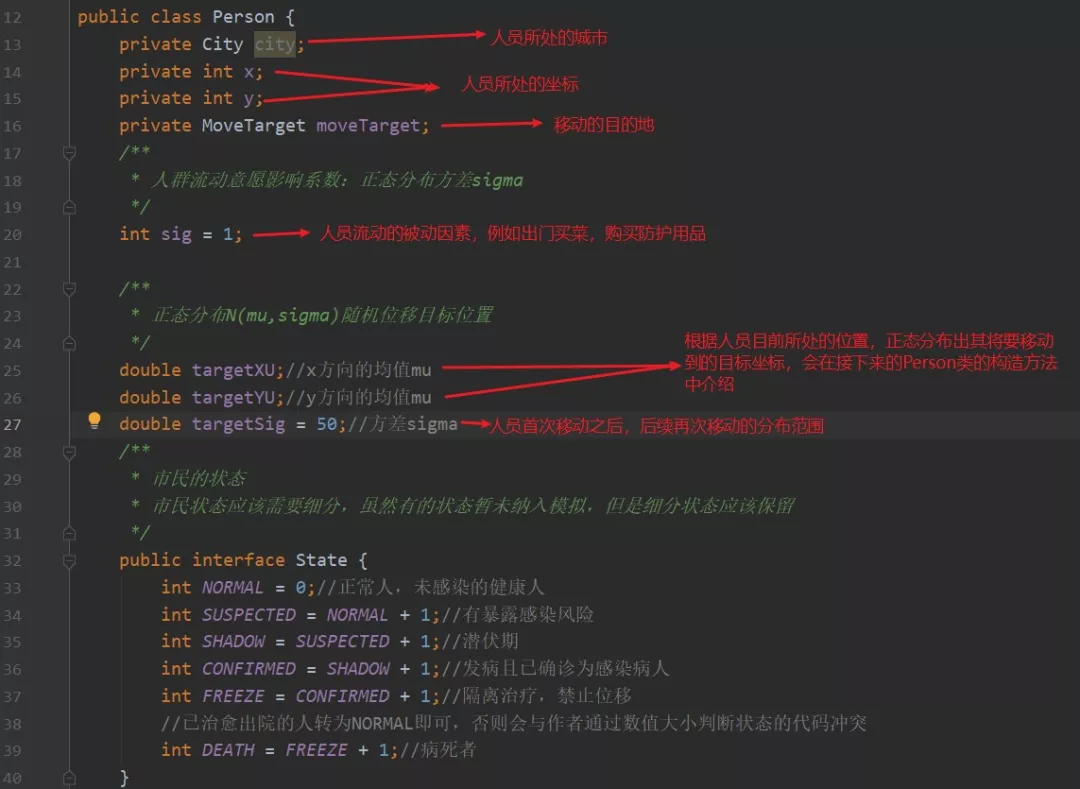Person的构造方法：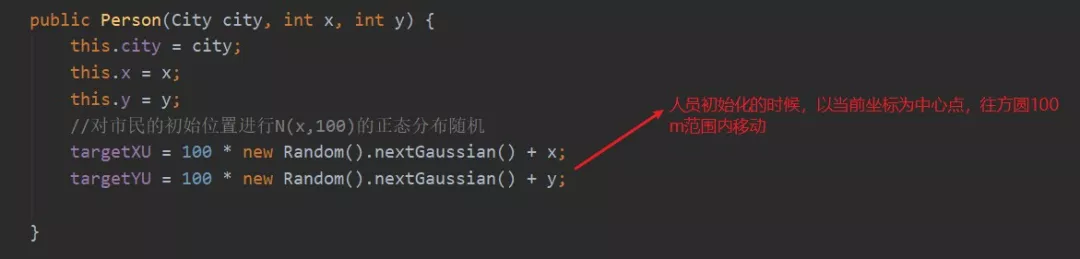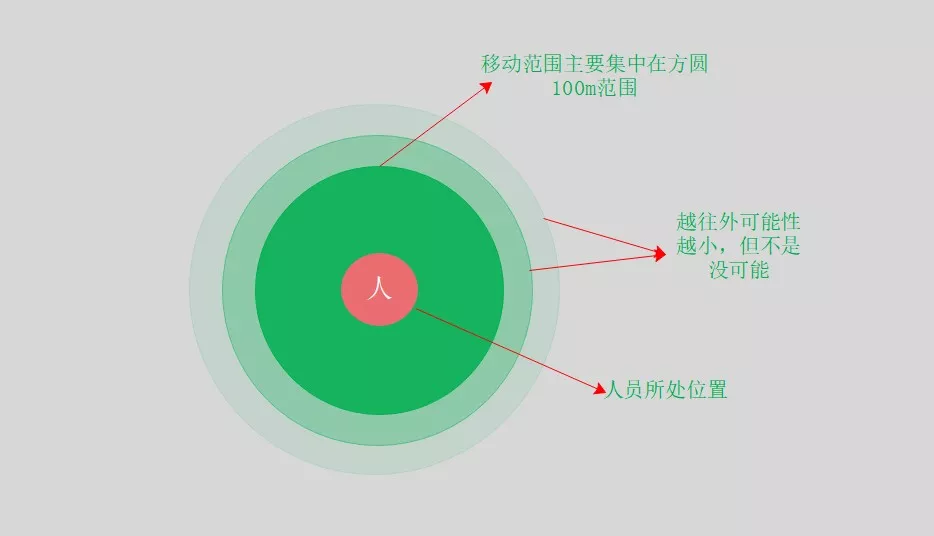Person类中的wantMove法的实现：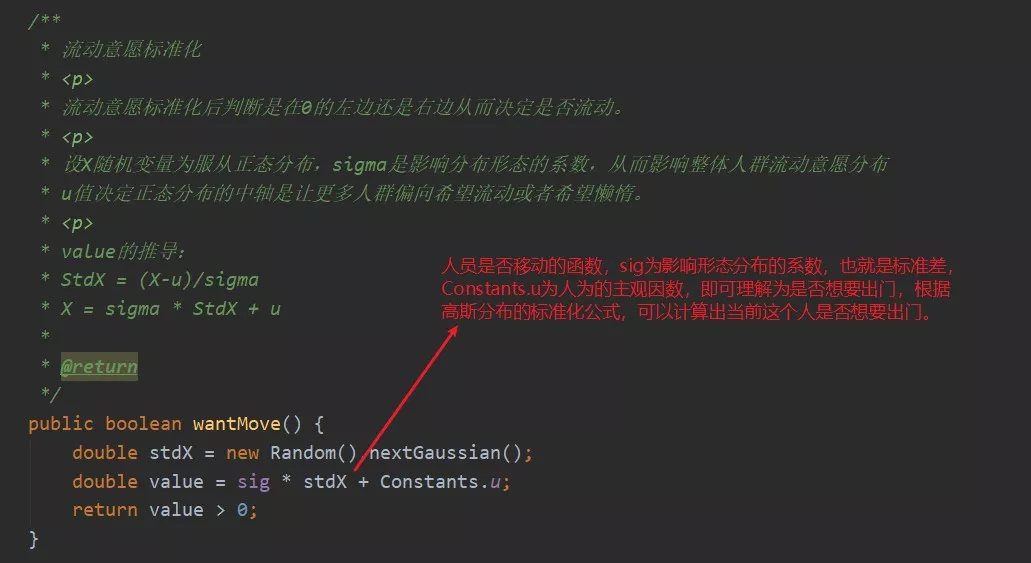Person 类中distance 方法的实现，用以判断是否能被感染：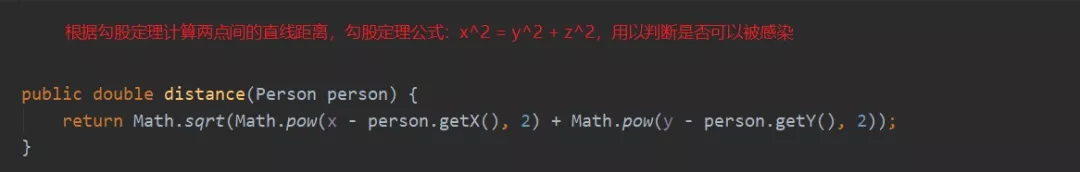## 3.5Person中的action方法

action方法是一个至关重要的方法，故单独提出为一个章节，该方法决定了用户坐标的移动，方法如下:

``````
/**

* 不同状态下的单个人实例运动行为

*/

private void action() {

if (state == State.FREEZE || state == State.DEATH) { return;

//如果处于隔离或者死亡状态，则无发行动

}

if (!wantMove()) {

//如果不想移动

return;

}

//存在流动意愿的，将进人流动，流动位移仍然遵循标准正态分布

if (moveTarget == null || moveTarget.isArrived()) {

// 如果人员没有目标的话，可能就是在家里呆烦了，他又想出⻔，那就在其目前移动的位置在随机移动

double targetX = targetSig * new Random().nextGaussian() + targetXU; double targetY = targetSig * new Random().nextGaussian() + targetYU;

// 最终想要到达的目的地

moveTarget = new MoveTarget((int) targetX, (int) targetY);

}

//计算运动位移

int dX = moveTarget.getX() - x; int dY = moveTarget.getY() - y;

// 勾股定理

double length = Math.sqrt(Math.pow(dX, 2) + Math.pow(dY, 2));//

if (length < 1) {

//判断是否到达目标点

moveTarget.setArrived(true); return;

}

/**

* 如果没有到达目标，一步一步的走，根据坐标方向，如果为正方向，就往前移动

1,

* 如果坐标轴的反方向，就移动

-1

* udx的结果只可能为两种三种情况：

-1 1 0

*/

int udX = (int) (dX / length);

//x轴移动步，符号为沿x轴前进方向

if (udX == 0 && dX != 0) {

if (dX > 0) {

udX = 1;

} else { udX = -1;

}

}

int udY = (int) (dY / length);

//y轴移动步，符号为沿x轴前进方向

if (udY == 0 && dY != 0) {

if (dY > 0) { udY = 1;

} else { udY = -1;

}

}

// 如果超过边界，就往回走

if (x > 700) {

//这个700也许是x方向边界的意思，因为画布大小

1000x800

//TODO:如果是边界那么似乎边界判断还差一个y方向

moveTarget = null;

if (udX > 0) { udX = -udX;

}

}
``````

## 3.6Person中的update方法

update方法是一个至关重要的方法，故单独提出为一个章节，该方法决定了根据用户不同的状态决定如何处理，方法如下:

``````/**

* 对各种状态的人进行不同的处理

*/

public void update() {

//@TODO找时间改为状态机

if (state == State.FREEZE || state == State.DEATH) { return;

//如果已经隔离或者死亡了，就不需要处理了

}

//处理已经确诊的感染者（即患者）

if (state == State.CONFIRMED && dieMoment == 0) {

int destiny = new Random().nextInt(10000)+1;

//命运数字[1,10000]随机数

if (1 <= destiny && destiny <= (int)(Constants.FATALITY_RATE * 10000))

{

//如果命运数字落在死亡区间

int dieTime = (int) (Constants.DIE_VARIANCE * new Random().nextGaussian()+Constants.DIE_TIME);

dieMoment = confirmedTime + dieTime;

//发病后确定死亡时刻

//System.out.printf("%d,%f,%d\n",destiny,Constants.FATALITY_RATE * 10000,dieTime);

}

else {

dieMoment = -1;

//逃过了死神的魔手

}

}

//*/

if (state == State.CONFIRMED && MyPanel.worldTime - confirmedTime >= Constants.HOSPITAL_RECEIVE_TIME) {

//如果患者已经确诊，且（世界时刻-确诊时刻）大于医院响应时间，即医院准备好病床了，可以抬走了

Bed bed = Hospital.getInstance().pickBed();

//查找空床位

if (bed == null) {

//没有床位了

// System.out.println("隔离区没有空床位");

} else {

//安置病人

state = State.FREEZE; x = bed.getX();

y = bed.getY(); bed.setEmpty(false);

}

}

//处理病死者

if((state == State.CONFIRMED || state == State.FREEZE )&& MyPanel.worldTime >= dieMoment && dieMoment > 0) {

state = State.DEATH;

//患者死亡

}

//处理发病的潜伏期感染者

state = State.CONFIRMED;

//潜伏者发病

confirmedTime = MyPanel.worldTime;

//刷新时间

}

//处理病死者

if((state == State.CONFIRMED || state == State.FREEZE )&& MyPanel.worldTime >= dieMoment && dieMoment > 0) {

state = State.DEATH;

//患者死亡

}

//处理发病的潜伏期感染者

state = State.CONFIRMED;

//潜伏者发病

confirmedTime = MyPanel.worldTime;

//刷新时间

}

//处理未隔离者的移动问题

action();

//处理健康⼈被感染的问题

List<Person> people = PersonPool.getInstance().personList; if (state >= State.SHADOW) {

return;

}

// 循环判断该⽤户是否会被其他人感染

for (Person person : people) {

//如果其他⼈为健康的，就继续判断下一个人

if (person.getState() == State.NORMAL) {

continue;

}

// 随机生成一个值

float random = new Random().nextFloat();

// 如果概率大于感染概率，并且小于安全距离，那么当前这个人肯定会被感染

if (random > Constants.BROAD_RATE && distance(person) < SAFE_DIST) { this.beInfected();

// 如果被感染了，就继续判断下一个

break;

}

}
}
``````